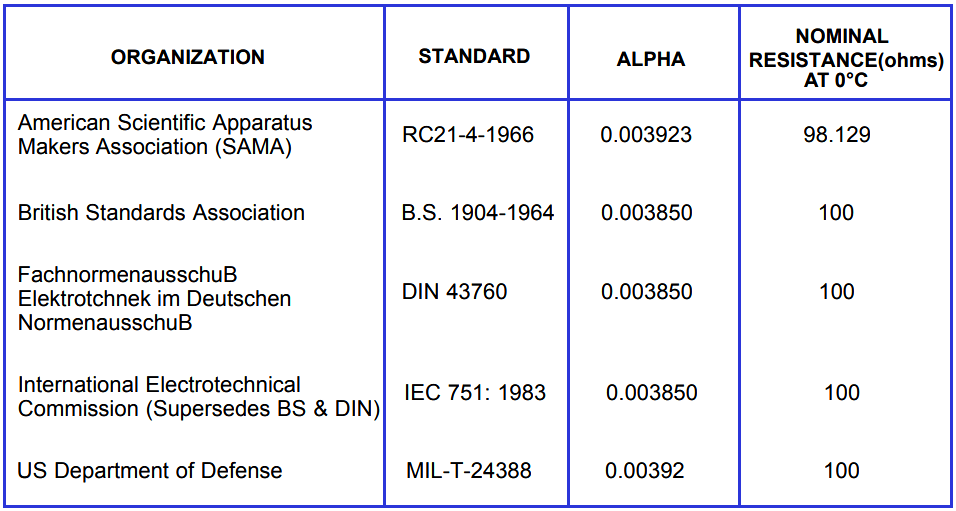# RTD Standards

RTD Standards

A resistance temperature detector or platinum resistance thermometer works on the principle that the electrical resistance of a metal changes in a significant and repeatable way when temperature changes. This resistance is inversely proportional to cross sectional area and proportional to length.

Platinum is the most widely used metal for resistance temperature detection due to the following characteristics:

1. chemical inertness
2. a temperature coefficient of resistance that is large enough to give readily measurable resistance changes with temperature
3. an almost strain free fabrication metal (in that resistance doesn’t drastically change with strain)
4. an almost linear relation between resistance and temperature

Each resistance versus temperature relation for an RTD is qualified by a term known as “alpha”. “Alpha” is the slope of the resistance between 0°C and 100°C. This is also referred to as the temperature coefficient of resistance, with the most common being 0.00385 Ω/Ω/°C.

Other types of RTD’s manufactured include copper, nickel and nickel alloys.

The amount of resistance of an individual RTD bulb (100Ω, 200Ω, etc.) is determined by the amount of metal between the terminal points and by the configuration of the element.

When ordering an RTD, the alpha and resistance value at 0°C (i.e.: Ro) must be specified to match the measuring instrumentation used with the RTD.

The RTD standard must also be specified. There are several RTD standards set by various organizations. These specifications are not identical and read out instrumentation must be adjusted for the specific standard of the RTD used with that equipment.

Differences in the alpha values of these standards can cause errors in measurement of an RTD if one standard is connected to the instrumentation of another standard.

The following chart indicates some common RTD standards.#### RTD Element TypesReference : gilsoneng.com

Be the first to get exclusive content straight to your email.
We promise not to spam you. You can unsubscribe at any time.

### 1 thought on “RTD Standards”

1.In general when we are at the field and does not have RTD table (i.e. Resistance vs temperature).

The general rule of thumb to know the temperature (in deg C) is 2.5 times the (actual resistance – 100) ohm.

E.g. 125 ohm is measured by RTD, then the temperature would be

===> 125-100 = 25
====> double the 25 => a) 50
====> half the 25 =>b) 12.5

Result is a)+b) ==>50+12.5 = ~ 62.5 Deg C would be the actual temperature.

To be very precise it would be 2.59 times the (actual resistance – 100) ohm.